161004 Epaper

 A Section B SectionC Section D Section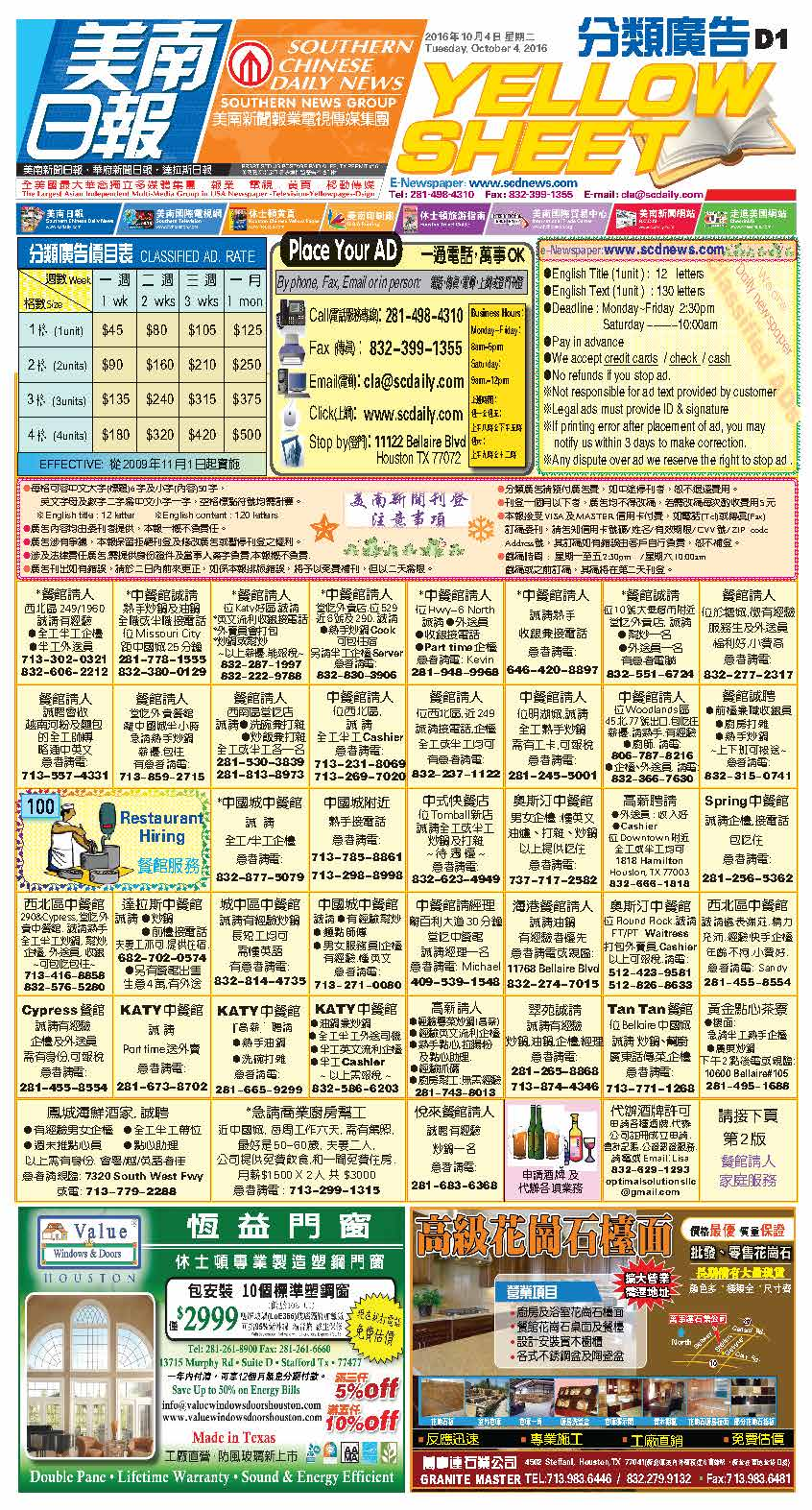161003 Epaper

 A Section B SectionC Section D Section161002 Epaper

 A Section B Section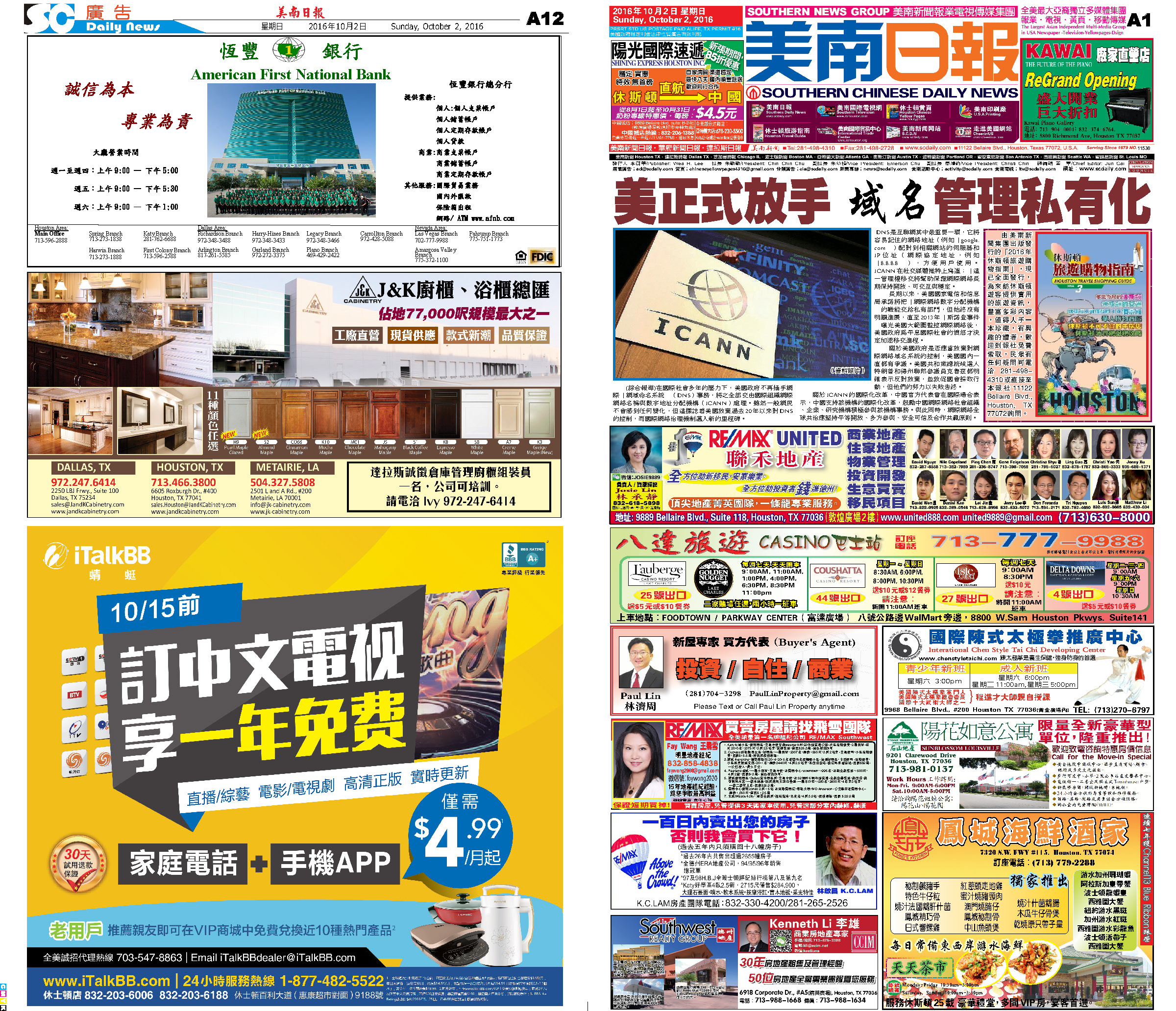C Section D Section161001 Epaper

 A Section B SectionC Section D Section160930 Epaper

 A Section B SectionC Section D Section160929 Epaper

 A Section B SectionC Section D Section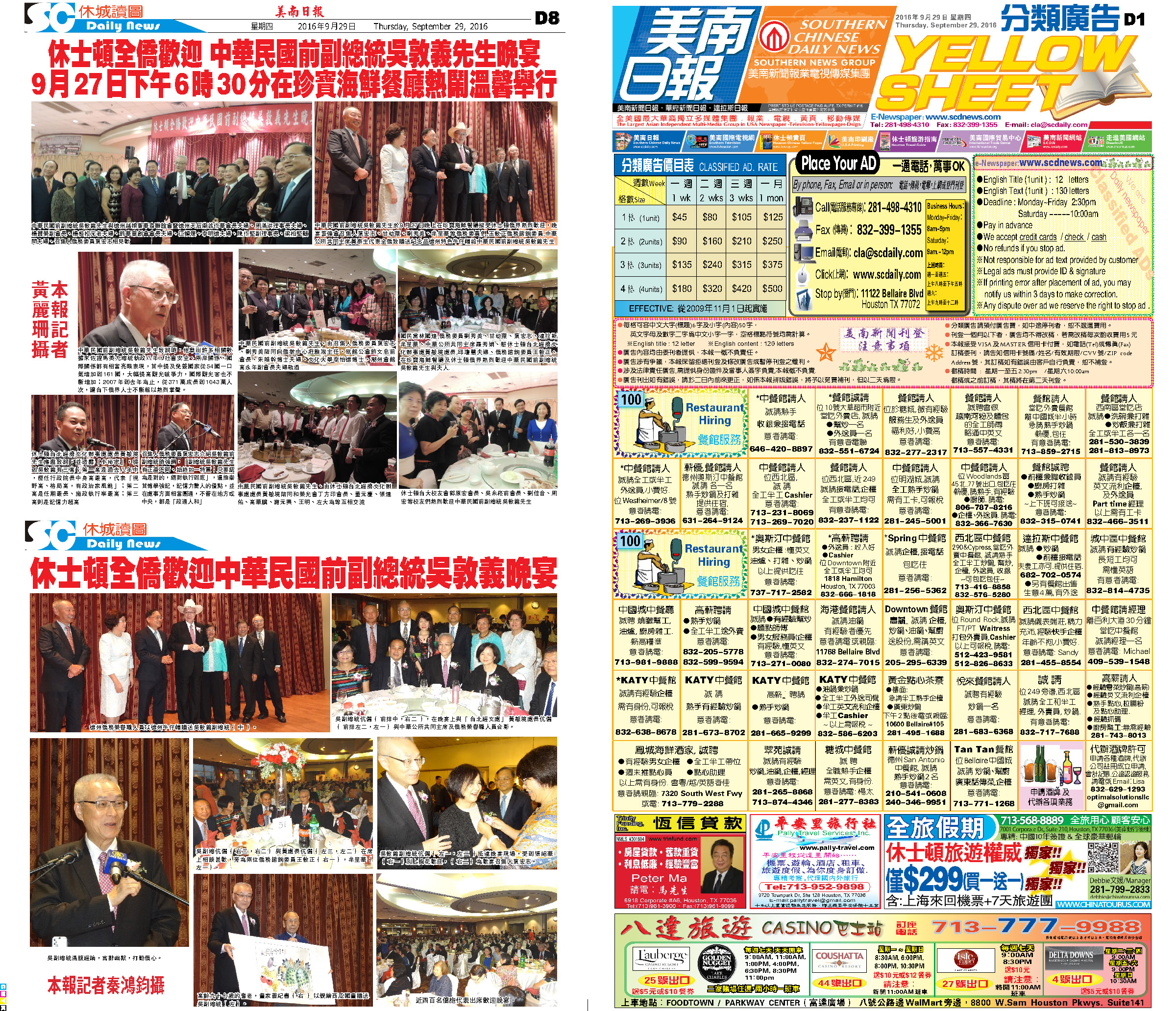160928 Epaper

 A Section B SectionC Section D Section160927 Epaper

 A Section B SectionC Section D Section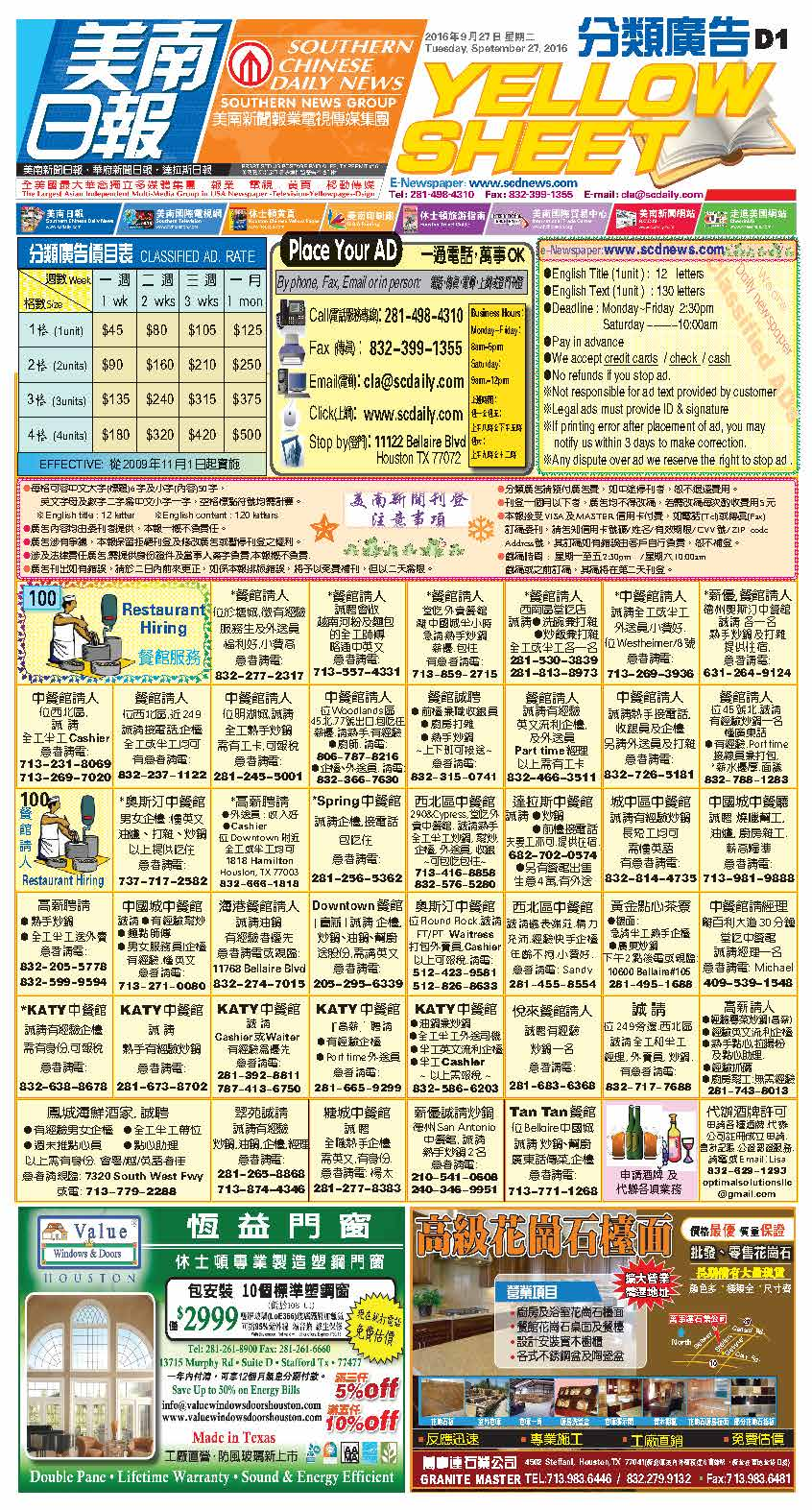160925 Epaper

 A Section B SectionC Section D Section160926 Epaper

 A Section B SectionC Section D Section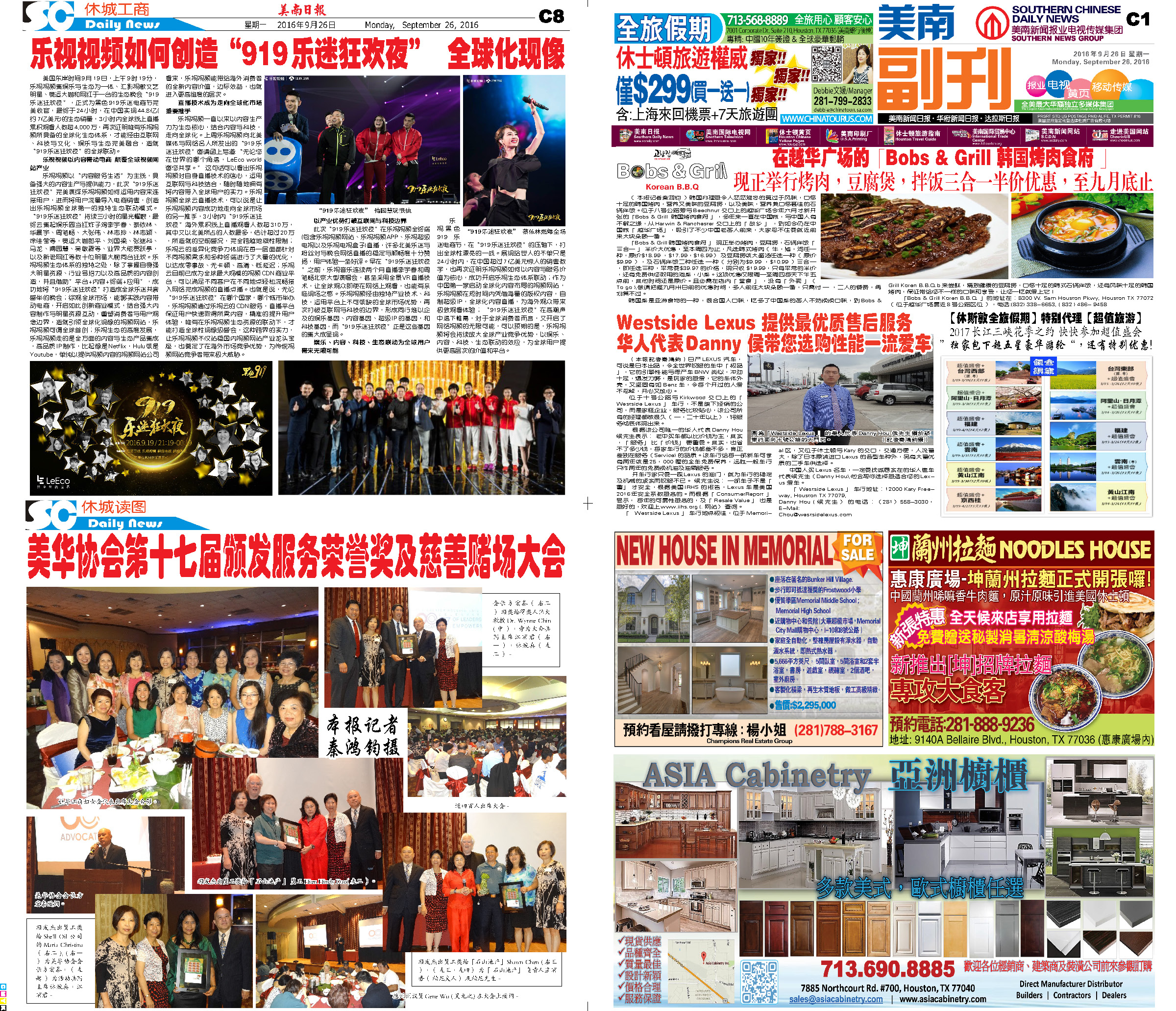160924 Epaper

 A Section B SectionC Section D Section